Related Articles

# How to create multiple CSV files from existing CSV file using Pandas ?

• Last Updated : 22 Jul, 2021

In this article, we will learn how to create multiple CSV files from existing CSV file using Pandas. When we enter our code into production, we will need to deal with editing our data files. Due to the large size of the data file, we will encounter more problems, so we divided this file into some small files based on some criteria like splitting into rows, columns, specific values of columns, etc.

First, let’s create a simple CSV file and use it for all examples below in the article. Create dataset using dataframe method of pandas and then save it to “Customers.csv” file or we can load existing dataset with the Pandas read_csv() function.

## Python3

 `import` `pandas as pd` `# initialise data dictionary.``data_dict ``=` `{``'CustomerID'``: [``1``, ``2``, ``3``, ``4``, ``5``, ``6``, ``7``, ``8``, ``9``, ``10``],``             ` `             ``'Gender'``: [``"Male"``, ``"Female"``, ``"Female"``, ``"Male"``,``                        ``"Male"``, ``"Female"``, ``"Male"``, ``"Male"``,``                        ``"Female"``, ``"Male"``],``             ` `             ``'Age'``: [``20``, ``21``, ``19``, ``18``, ``25``, ``26``, ``32``, ``41``, ``20``, ``19``],``             ` `             ``'Annual Income(k\$)'``: [``10``, ``20``, ``30``, ``10``, ``25``, ``60``, ``70``,``                                   ``15``, ``21``, ``22``],``             ` `             ``'Spending Score'``: [``30``, ``50``, ``48``, ``84``, ``90``, ``65``, ``32``, ``46``,``                                ``12``, ``56``]}` `# Create DataFrame``data ``=` `pd.DataFrame(data_dict)` `# Write to CSV file``data.to_csv(``"Customers.csv"``)` `# Print the output.``print``(data)`

Output: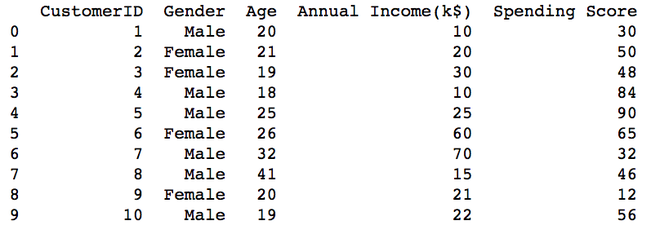## Creating multiple  CSV files from the existing CSV file

To do our work, we will discuss different methods that are as follows:

### Method 1: Splitting based on rows

In this method, we will split one CSV file into multiple CSVs based on rows.

## Python3

 `import` `pandas as pd` `# read DataFrame``data ``=` `pd.read_csv(``"Customers.csv"``)` `# no of csv files with row size``k ``=` `2``size ``=` `5` `for` `i ``in` `range``(k):``    ``df ``=` `data[size``*``i:size``*``(i``+``1``)]``    ``df.to_csv(f``'Customers_{i+1}.csv'``, index``=``False``)` `df_1 ``=` `pd.read_csv(``"Customers_1.csv"``)``print``(df_1)` `df_2 ``=` `pd.read_csv(``"Customers_2.csv"``)``print``(df_2)`

Output: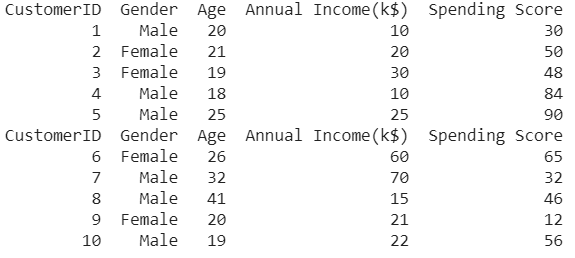### Method 2: Splitting based on columns

Example 1:

Using groupby() method of Pandas we can create multiple CSV files. To create a file we can use the to_csv() method of  Pandas. Here created two files based on “male” and “female” values of Gender columns.

## Python3

 `import` `pandas as pd` `# read DataFrame``data ``=` `pd.read_csv(``"Customers.csv"``)` `for` `(gender), group ``in` `data.groupby([``'Gender'``]):``     ``group.to_csv(f``'{gender}.csv'``, index``=``False``)` `print``(pd.read_csv(``"Male.csv"``))``print``(pd.read_csv(``"Female.csv"``))`

Output: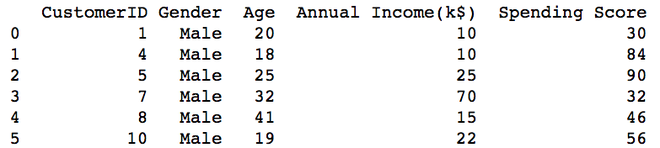Male.csv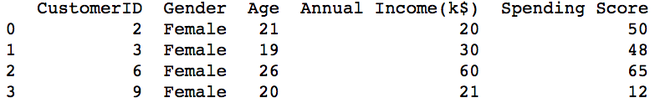Female.csv

Example 2:

We can group more than two columns and can create multiple files on the basis of a combination of unique values from both Columns value. Take Gender and Annual Income columns.

## Python3

 `import` `pandas as pd` `# read DataFrame``data ``=` `pd.read_csv(``"Customers.csv"``)` `for` `(Gender, Income), group ``in` `data.groupby([``'Gender'``, ``'Annual Income(k\$)'``]):``    ``group.to_csv(f``'{Gender} {Income}.csv'``, index``=``False``)``    ``print``(pd.read_csv(f``'{Gender} {Income}.csv'``))`

Output: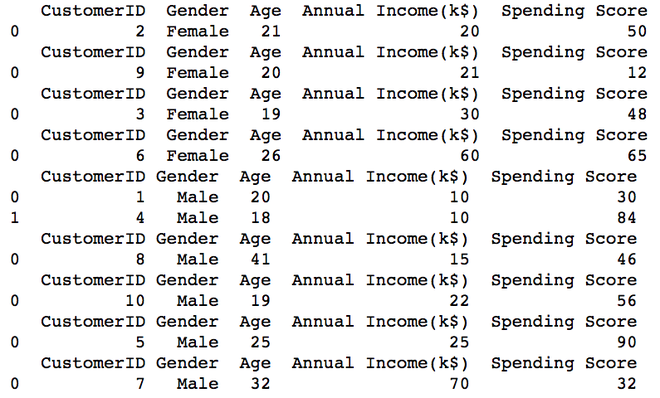All Nine CSV files

Example 3:

We will filter the columns based on the specific column name Gender to its values (Male and Female). Then convert that to CSV file using to_csv in pandas.

## Python3

 `import` `pandas as pd` `# read DataFrame``data ``=` `pd.read_csv(``"Customers.csv"``)` `male ``=` `data[data[``'Gender'``] ``=``=` `'Male'``]``female ``=` `data[data[``'Gender'``] ``=``=` `'Female'``]` `male.to_csv(``'Gender_male.csv'``, index``=``False``)``female.to_csv(``'Gender_female.csv'``, index``=``False``)` `print``(pd.read_csv(``"Gender_male.csv"``))``print``(pd.read_csv(``"Gender_female.csv"``))`

Output: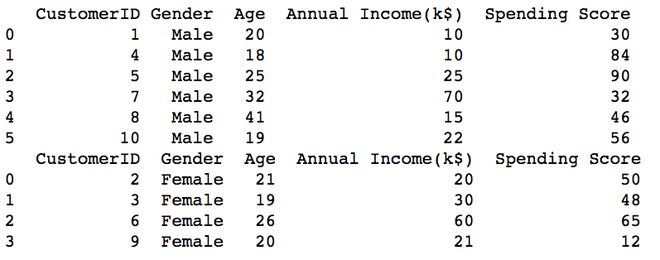### Method 3: Splitting based both on Rows and Columns

Using groupby() method of Pandas we can create multiple CSV files row-wise. To create a file we can use the to_csv() method of Pandas. Here created two files based on row values “male” and “female” values of specific Gender column for Spending Score.

## Python3

 `for` `(gender), group ``in` `data[``'Spending Score'``].groupby(data[``'Gender'``]):``    ``group.to_csv(f``'{gender}Score.csv'``, index``=``False``)``    ` `print``(pd.read_csv(``"MaleScore.csv"``))``print``(pd.read_csv(``"FemaleScore.csv"``))`

Output: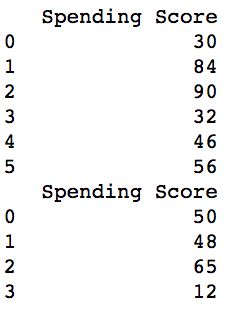Attention geek! Strengthen your foundations with the Python Programming Foundation Course and learn the basics.

To begin with, your interview preparations Enhance your Data Structures concepts with the Python DS Course. And to begin with your Machine Learning Journey, join the Machine Learning – Basic Level Course

My Personal Notes arrow_drop_up